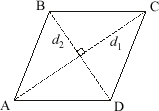# rhombus

Rhombus

A parallelogram with four congruentsides. Note that the diagonals of a rhombus are perpendicular (as is the case with all kites).

Note: A square is a special kind of rhombus.

 Rhombuss = side length of rhombush = height of rhombusd1 = long diagonal of rhombusd2 = short diagonal of rhombus Area = hs         = s2 sin A         = s2 sin B         = (½)d1d2